Courses

# NCERT Solutions Chapter 11 - Constructions (I), Class 9, Maths Class 9 Notes | EduRev

Created by: Indu Gupta

## Class 9 : NCERT Solutions Chapter 11 - Constructions (I), Class 9, Maths Class 9 Notes | EduRev

The document NCERT Solutions Chapter 11 - Constructions (I), Class 9, Maths Class 9 Notes | EduRev is a part of Class 9 category.
All you need of Class 9 at this link: Class 9

Exercise 11.1

1. Construct an angle of 90° at the initial point of a given ray and justify the construction.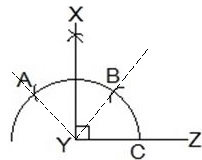Steps of construction:

Step 1: A ray YZ is drawn.
Step 2: With Y as a centre and any radius, an arc ABC is drawn cutting YZ at C.
Step 3: With C as a centre and the same radius, mark a point B on the arc ABC.
Step 4: With B as a centre and the same radius, mark a point A on the arc ABC.
Step 5: With A and B as centre, draw two arcs intersecting each other with the same radius at X.
Step 6: X and Y are joined and a ray XY making an angle 90° with YZ is formed.

Justification for construction:
We constructed ∠BYZ = 60° and also ∠AYB = 60°.
Thus, ∠AYZ = 120°.
Also, bisector of ∠AYB is constructed such that:
∠AYB = ∠XYA + ∠XYB
⇒ ∠XYB = 1/2∠AYB
⇒ ∠XYB = 1/2×60°
⇒ ∠XYB = 30°
Now,
∠XYZ = ∠BYZ + ∠XYB = 60° + 30° = 90°

2. Construct an angle of 45° at the initial point of a given ray and justify the construction.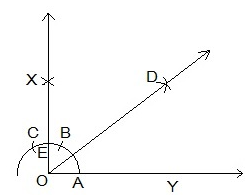Steps of construction:

Step 1: A ray OY is drawn.
Step 2: With O as a centre and any radius, an arc ABC is drawn cutting OY at A.
Step 3: With A as a centre and the same radius, mark a point B on the arc ABC.
Step 4: With B as a centre and the same radius, mark a point C on the arc ABC.
Step 5: With A and B as centre, draw two arcs intersecting each other with the same radius at X.
Step 6: X and Y are joined and a ray making an angle 90° with YZ is formed.
Step 7: With A and E as centres, two arcs are marked intersecting each other at D and the bisector of ∠XOY is drawn.

Justification for construction:
By construction,
∠XOY = 90°
We constructed the bisector of ∠XOY as DOY.
Thus,
∠DOY = 1/2 ∠XOY
∠DOY = 1/2×90° = 45°

3. Construct the angles of the following measurements:
(i) 30°

(ii) 22.5°

(iii) 15°

(i) 30°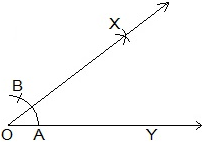Steps of constructions:
Step 1: A ray OY is drawn.
Step 2: With O as a centre and any radius, an arc AB is drawn cutting OY at A.
Step 3: With A and B as centres, two arcs are marked intersecting each other at X and the bisector of is drawn.
Thus, ∠XOY is the required angle making 30° with OY.

(ii) 22.5°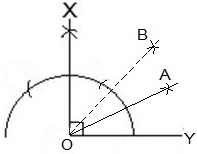Steps of constructions:
Step 1: An angle ∠XOY = 90° is drawn.
Step 2: Bisector of ∠XOY is drawn such that ∠BOY = 45° is constructed.
Step 3: Again, ∠BOY is bisected such that ∠AOY is formed.
Thus, ∠AOY is the required angle making 22.5° with OY.

(iii) 15°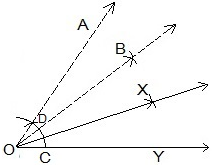Steps of constructions:
Step 1: An angle ∠AOY = 60° is drawn.
Step 2: Bisector of ∠AOY is drawn such that ∠BOY = 30° is constructed.
Step 3: With C and D as centres, two arcs are marked intersecting each other at X and the bisector of ∠BOY is drawn.
Thus, ∠XOY is the required angle making 15° with OY.

4. Construct the following angles and verify by measuring them by a protractor:
(i) 75°         (ii) 105°        (iii) 135°

(i) 75°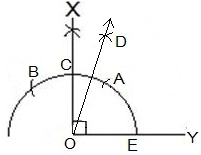Steps of constructions:

Step 1: A ray OY is drawn.
Step 2: An arc BAE is drawn with O as a centre.
Step 3: With E as a centre, two arcs are A and C are made on the arc BAE.
Step 4: With A and B as centres, arcs are made to intersect at X and ∠XOY = 90° is made.
Step 5: With A and C as centres, arcs are made to intersect at D
Step 6: OD is joined and and ∠DOY = 75° is constructed.
Thus, ∠DOY is the required angle making 75° with OY.

(ii) 105°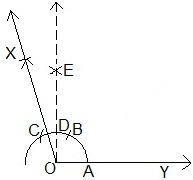Steps of constructions:

Step 1: A ray OY is drawn.
Step 2: An arc ABC is drawn with O as a centre.
Step 3: With A as a centre, two arcs are B and C are made on the arc ABC.
Step 4: With B and C as centres, arcs are made to intersect at E and ∠EOY = 90° is made.
Step 5: With B and C as centres, arcs are made to intersect at X
Step 6: OX is joined and and ∠XOY = 105° is constructed.
Thus, ∠XOY is the required angle making 105° with OY.

(iii) 135°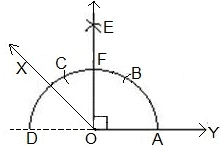Steps of constructions:Step 1: A ray DY is drawn.
Step 2: An arc ACD is drawn with O as a centre.
Step 3: With A as a centre, two arcs are B and C are made on the arc ACD.
Step 4: With B and C as centres, arcs are made to intersect at E and ∠EOY = 90° is made.
Step 5: With F and D as centres, arcs are made to intersect at X or bisector of ∠EOD is constructed.
Step 6: OX is joined and and ∠XOY = 135° is constructed.
Thus, ∠XOY is the required angle making 135° with DY.

5. Construct an equilateral triangle, given its side and justify the construction.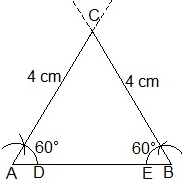Steps of constructions:
Step 1: A line segment AB=4 cm is drawn.
Step 2: With A and B as centres, two arcs are made.
Step 4: With D and E as centres, arcs are made to cut the previous arc respectively and forming angle of 60° each.
Step 5: Lines from A and B are extended to meet each other at C.
Thus, ABC is the required triangle formed.

Justification:
By construction,
AB = 4 cm, ∠A = 60° and ∠B = 60°
We know that,
∠A + ∠B + ∠C = 180° (Sum of the angles of a triangle)
⇒ 60° + 60° + ∠C = 180°
⇒ 120° + ∠C = 180°
⇒ ∠C = 60°
BC = CA = 4 cm (Sides opposite to equal angles are equal)
AB = BC = CA = 4 cm
∠A = ∠B = ∠C = 60°

Offer running on EduRev: Apply code STAYHOME200 to get INR 200 off on our premium plan EduRev Infinity!

,

,

,

,

,

,

,

,

,

,

,

,

,

,

,

,

,

,

,

,

,

,

,

,

,

,

,

;